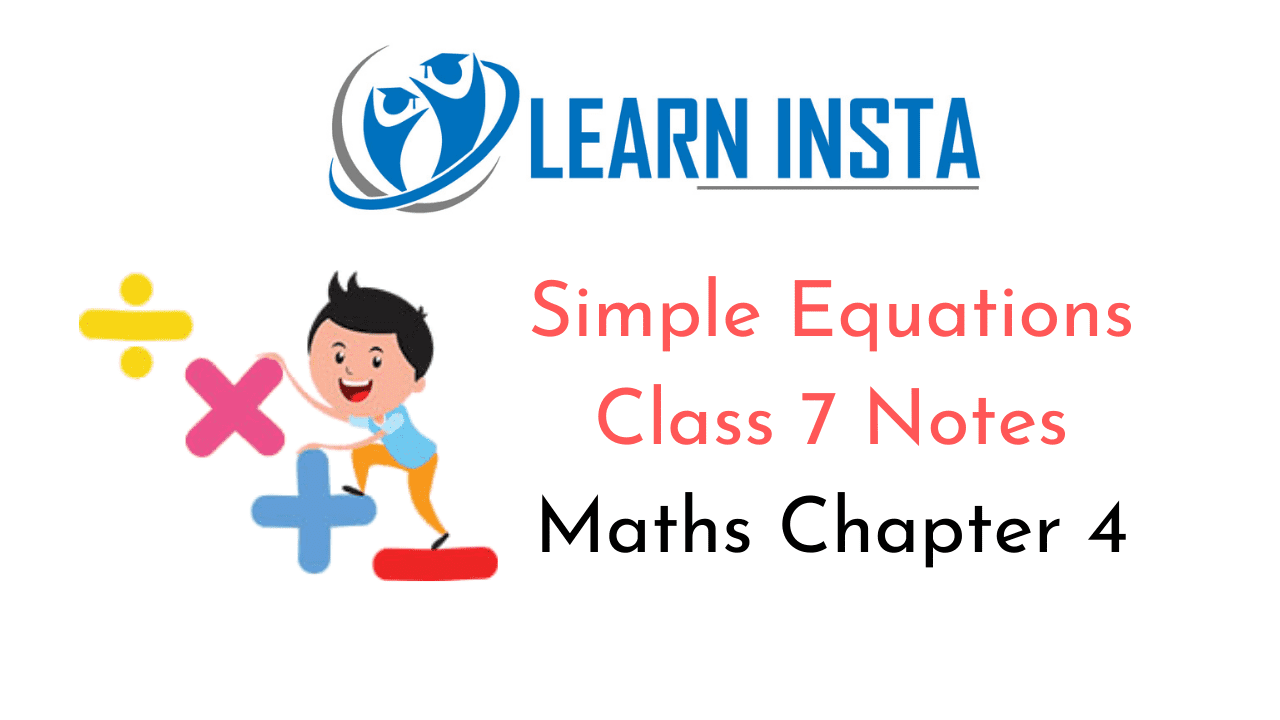On this page, you will find Simple Equations Class 7 Notes Maths Chapter 4 Pdf free download. CBSE NCERT Class 7 Maths Notes Chapter 4 Simple Equations will seemingly help them to revise the important concepts in less time.

## CBSE Class 7 Maths Chapter 4 Notes Simple Equations

### Simple Equations Class 7 Notes Conceptual Facts

1. An equation having only one variable with highest power 1 is called linear equation.
For example:
3x – 5 = 11 with 1 variable x
5 – 2y = 0 with 1 variable y

2. A number which satisfies the given linear equation is called its solutions.
For example: 3x – 2 = 4 requires a particular value of x which satisfies this equation and that value of x will be the solution of the equation.

3. Rules for finding the solution of linear equations:
(i) Trial and error method:
Let us take an equation, 3x-2 = 4
Put x = 1 inLHS, 3 x 1-2=3 – 2 ≠ 4 RHS
Put x = 2 in LHS, 3 x 2 – 2=6-2=4 RHS
Thus, by putting x = 2, we get RHS
Hence, x = 2 is the solution of the given equation.

(ii) Transposition method: A term may be transposed from one side of the equation to the other side with the change its sign.
For example:
(a) 3x – 6 = 9 ⇒ 3x = 6 + 9 (Transposing -6 from LHS to RHS by changing its sign)

(b) 3x-5=x ⇒ 3x-x = 5 (Transposingx from RHS to LHS and 5 from LHS to RHS by changing their signs)
multiplying or dividing on both sides

(iii) Adding, subtracting, multiplying or dividing on both sides
For example:
(a) 3x – 10 – 5 ⇒ 3x – 10+ 10 = 5 + 10 ⇒ 3x = 15 (Adding 10 to both sides)
(b) 5x + 12 = 27 ⇒ 5x + 12- 12 = 27 – 12 ⇒ 5x = 15 (Subtracting 12 from both sidés)
(c) $$\frac{x}{5}$$ = 10 ⇒ $$\frac{x}{5}$$ x 5 =10 x 5 ⇒ x=50 (Multiplyingbothsidesby5)
(d) 3x = 18 ⇒ 3x + 3 = 18÷ 3 (Dividing both sides by 3)
⇒ x = 6